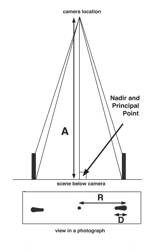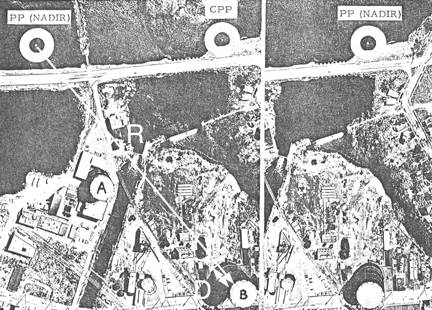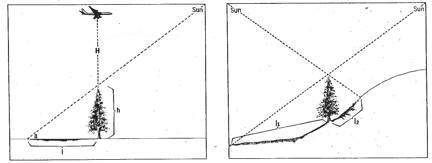Basic Photogrammetry Techniques Andrew Davidhazy Imaging and Photographic Technology School of Photographic Arts and Sciences Rochester Institute of Technology Measuring Heights of Displaced Objects   The exaggerated displacement of tall objects pictured near the edges of large- scale, vertical photographs sometimes permits accurate measurement of object heights on single prints. This specialized technique of height evaluation is feasible provided that:   a. The principal point can be accepted as the nadir position. b. The flight altitude above the base of the object can be precisely determined. c. Both the top and base of the object are clearly visible and the top is directly above the     bottom. d. The degree of image displacement is great enough to be accurately measured with      available equipment, e.g., an engineer's scale.When all these conditions can be met, object heights may be determined by this relationship:                                                 D x A         Height (in feet) =  ------------------                                                  R   Where D is the length of the displaced image in inches. A is the altitude above the base of the object in feet.  R is the radial distance from the nadir to the top of the displaced image, in inches.   The NADIR point on a vertical aerial photograph is located where the optical axis (or roughly the center) of a photograph is. This can be approximately found by drawing diagonal lines from the corners of the photo and where they intersect that is the middle.   Practical application of this method can be illustrated by use of the figures below. These photographs were taken from a flight altitude of 3,000 feet with a camera focal length of 6 inches. Radial measurements from the assumed nadir position to tanks" A" and "B" may be substituted in the preceding formula:   Height (Tank "A") = 0.18" x 3,000' / 2. 34" = 231 feet   Height (Tank "B") = 0.38" x 3,000' / 5.00" =  228 feet   Thus both tanks are about the same height, and the measurement of tank "B" could be re-checked on the right-hand photograph:   Height (Tank "B") = O. 30" x 3,000 / 3.84" =  234 feet   In these examples, the three estimates of height show closer agreement than may be obtained by casual measurement. In the last computation, for instance, an error of only 0. 02-inch in the length of the displaced image would have affected the final answer by 16 feet.Determining the Size of Irregular Areas by Weight   The total area of several irregularly shaped areas of the same scale can be determined quite accurately by the following steps,   Step 1. Weigh the photos on which the areas of interest are pictured.   Step 2. Cut out the irregularly shaped areas from the photographs. Weigh the cut‑out section very accurately.   Step 3. Cut out an area of known size, and weigh it very accurately.   Step 4. Divide the weight of the representative area into the weight of the cut‑out sections of the area to be found.   Step 5. As an accuracy check, add the weights found in Steps 2 and 3 above. Their total should equal the weight found in Step 1.   This method requires that an extremely accurate scale (for measurement of weight) be available, and that ground elevations of both the irregularly shaped and the representative areas all be the same, since photo scale (and area) changes with the altitude of the terrain. This method is very good for measurement of water areas, because the surface areas are level and usually relatively close to the same elevation.   Application: To find the size of an area which has been flooded, for example, the following should be done:   1. Cut out the flooded areas from the photos, and weigh them. They are found to weigh 8,000 grams.   2. Cut out a representative area. An area of 640 acres (one square mile, or one section) is selected, and found to weigh 800 grams.   3. Then 8000 g / 800 g = 10 and thus the total flooded area is 10 sq mi     Determining Graphically the Size of Irregular Areas by Approximation     The total area of irregularly shaped subject areas reproduced in a photograph at the same scale can be determined graphically quite accurately by the following steps:   Step 1. On the print find the area of a known quadrangle based on "ground truth" or some other method by which the scale of the photograph can be determined.   Step 2. Project this figure al over the image of  the subject whose area needs to be determined. Instead of drawing directly on the print you could draw on an acetate overlay.   Step 3. Count the number of whole quadrangles that can be identified within the confines of the subject boundaries whose area needs to be determined.   Step 4. Estimate the total number of whole quadrangles that would be made up by those that only partially fall within the boundaries of the subject area.   Step 4. Add the number of whole quadrangles to the area comprised by the partial quadrangles.   Step 6. Multiply the total number of quadrangles by the area of the reference quadrangle. This is the approximate area of the subject of interest.       Determining Subject Height from Shadows     The calculation of object height from its shadow length requires that:   1. The exact "sun" or sidereal time when the photograph was taken be known. 2. The precise geographic location of the object be known. 3. A table of sun declinations at different times throughout a four-year period be available. The table below is sufficiently accurate for most calculations. The appropriate formula is:                                            (H) ( 1) (tan solar altitude)                                h = --------------------------------------                                                         f where   h = height of the object H = altitude of the photo plane above the ground, l = length of the shadow f = focal length of the camera.   The solar altitude in any location at any given time can be obtained from various government agencies concerned with astronomy and time. It can also be computed by using the following factors:   1. The sun's declination on the day of the photography (obtainable from table 1) (a) 2. The latitude of the area. (b) 3. The difference in longitude between the celestial meridian passing through the sun at the instant of exposure and the meridian of the area which is imaged. (c)   When these qualities are known, the solar altitude can be computed by the relation:                        Solar altitude =  sin x = (cos a) (cos b) (cos c) + (sin a) (sin b)   The relationship of shadow length and object height to the altitude of the sun are obvious, as shown in Figure 1. It is equally obvious, however, that this method may lead to serious errors if shadows do not fall on level ground (or other flat surface). If the ground slopes down, shadows will appear too long. If the ground slopes up, shadows will appear too short. Shadows that can be seen falling water surface usually provide a very good reference plane.   Alternatively, if the sun's altitude above the horizon is already known, a short cut method of determining the height of a vertical subject above level ground can be found by referring to the figure below on the left.The height of vertical objects can be calculated accurately from vertical photographs by measurements made from a photograph regardless of its scale of reproduction simply based on shadow length if location, sun altitude, camera focal length, and flying height are known. Use the following formula already given above:                                                                          H l  (tan a)                                                              h = ----------------                                                                               f   where h is the object height, H is the flight altitude above the base of the subject, 1 is the shadow length, a is the altitude of the sun, and f is the camera focal length, as shown on the left. As shown on the right, however, shadows must fall on level ground. If the surface slopes down, shadows will appear too long. If the surface slopes up, shadows will appear too short.   These guidelines were copied from booklets and pamphlets whose origin I can't remember. All are over 30 years old. If they are your writing and you'd like me to delete them from here please let me know and the section will be eliminated ASAP. The bit on quadrangles and use as the basis for area measurement I made up "off the top of my head" and so look at it with suspicion. Happy measuring!! Andrew Davidhazy, andpph@rit.edu